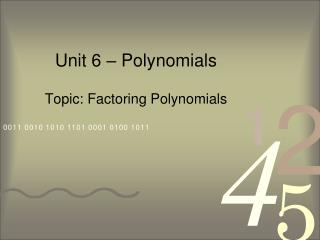DownloadDownload PresentationUnit 6 – Polynomials

# Unit 6 – Polynomials

Télécharger la présentation## Unit 6 – Polynomials

- - - - - - - - - - - - - - - - - - - - - - - - - - - E N D - - - - - - - - - - - - - - - - - - - - - - - - - - -
##### Presentation Transcript

1. Unit 6 – Polynomials Topic: Factoring Polynomials

2. Factoring Things to Remember • ALWAYS look for a GCF first! • 4-term polynomials: factor by grouping or Punnett Square. • 3-term polynomials: where factoring is concerned, these will ALWAYS be quadratics. • 2-term polynomials: look for difference of squares, sum of cubes, or difference of cubes; each one has its own rule for factoring. • CHECK YOUR ANSWER: your final factors should multiply back out to become your original expression.

3. Factoring Polynomials By Grouping • Factor the following completely. Group the first two terms & the last two terms. The – must stay attached to the 9, but the problem is still addition/subtraction. Now factor out a GCF from each group. We want each binomial to be identical, so we factor out –9 instead of 9. The repeated binomial is one factor, the two terms we took out make up the second factor. We’re not quite done. The second factor is a difference of squares, so we must continue factoring. We could also factor using our friend the Algebraic Punnett Square.

4. Factoring Polynomials Using the Square Remember factoring is working backwards. These terms will go inside the square, so we need 4 boxes. • Factor the following completely. Now we find factors of each term to place outside the square. Remember it’s easiest to start with the constant (in this case 18). Now we have two factors. Again one is a difference of squares so we must factor it further. Hey, we got the same answer as the previous slide!

5. Sum & Difference of Cubes (put these on a note card if you didn’t last semester) • Sum of two cubes • Binomial sum of two perfect cubes. • Factors as follows: • Difference of two cubes • Binomial difference of two perfect cubes. • Factors as follows:

6. Factoring Sum & Difference of Cubes 8x3& 27 are perfect cubes. Rewrite the binomial using those cubes. • Factor the following completely. Apply the rule for difference of cubes & simplify; replace x in the formula with 2x, replace y with 3.

7. Factoring Sum & Difference of Cubes This isn’t a cubic function at all, but we can factor out a GCF… • Factor the following completely. …now we have a sum of cubes. Rewrite the binomial & factor using the appropriate rule. Don’t forget that the 5x must remain as a factor.

8. JOURNAL ENTRY • TITLE: Checking My Understanding: Factoring Polynomials • Review your notes from this presentation & create and complete the following subheadings in your journal: • “Things I already knew:” Identify any information with which you were already familiar. • “New things I learned:” Identify any new information that you now understand. • “Questions I still have:” What do you still want to know or do not fully understand?

9. Homework • Textbook Section 6-4 (pg. 433): 16, 20-31, 33-39 • Should be complete by 1/27